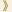HomeShaw Laureates2008Mathematical SciencesEssay

Mathematics and Physics have, over the centuries, had a long and close relationship. The modern era was ushered in by Galileo who said that the laws of nature were written in the language of mathematics. This was taken a giant step forward by Isaac Newton who developed and applied calculus to the study of dynamics. From that time on the whole theoretical framework of physics has been formulated in terms of differential equations.

Both of the 2008 Shaw Laureates in the Mathematical Sciences,Vladimir Arnold and Ludwig Faddeev, are part of this great tradition. Arnold's contributions are mainly in classical mechanics, emphasizing the geometrical aspects as developed over the centuries by Newton, Riemann and Poincare. Faddeev has been attracted more by the challenges of quantum theory and the algebraic formalism that is related to it.

Arnold has made many important contributions to a wide variety of problems on the Analysis/geometry frontier, but his most famous is the Kolmogorov-Arnold- Möser (KAM) theory. This theory shows the persistence of quasi-periodic orbits of dynamical systems under suitable perturbations. Originating in Newton's work on a single planetary orbit, it deals with the more general N-body problem, has been enormously influential and has important applications from the solar system to particle accelerators. Arnold also pointed out (in 1964) a subtle instability, now called Arnold diffusion, which has been much studied by mathematicians and physicists.

The general theory of Hamiltonian mechanics (in which energy is conserved) has an elegant formulation in geometrical terms – symplectic geometry. Arnold made a deep study of this subject and formulated some profound conjectures relating Hamiltonian flows to topology. These were very influential, leading to a fruitful development over the subsequent decades, culminating in the proof of some versions of the Arnold conjectures by Andreas Floer and others.

Geometrical structures frequently exhibit singularities, a subject of study by algebraists and geometers for a long time, but Arnold's interest in them centres around their appearance as caustics in wave-propagation. He emphasized the geometrical approach in this field but incorporated new results from algebra and topology.

Arnold was also a pioneer of the geometric approach to the study of the Euler equations for the dynamics of ideal fluids, an approach that has had great influence over the last thirty years. In 1966 he obtained general criteria for the stability, both linear and non-linear, of the Euler equations, while in 1974 he provided an interpretation of the helicity invariant of the Euler equations as an asymptotic Hopf invariant of linked vortex lines.

Another connection between geometry and analysis emerges from real algebraic geometry associated with the characteristics of partial differential equations. Whereas complex algebraic geometry has a rich and beautiful theory, most of this disappears over the real numbers. For plane curves one of Hilbert's famous problems asked questions about the disposition of real ovals. Arnold attacked this problem by a highly ingenious and effective topological approach giving the best results at the time.

Ludwig Faddeev has made many important contributions to quantum physics and to the differential equations that underpin it.

He is best known for his work with Victor Popov showing the right way to quantize the non-Abelian gauge theories which underlie all contemporary work on sub-atomic physics. Gauge symmetry is well understood in electromagnetic theory as formulated by Clerk Maxwell: it amounts to the ambiguity of the vector potential. The challenge was how to extend this simple linear situation to the non-linear case of the Yang-Mills equations relevant to particle physics. The answer lay in the introduction of what are now called "Faddeev - Popov ghosts". These have totally transformed the theory in an elegant and conceptual way, leading in due course to the work of 't Hooft and Veltman which was recognized by the Nobel Prize for Physics of 1999.

One of the surprising results of the past fifty years has been the discovery that a number of interesting non-linear partial differential equations that arise, in certain simplified physical situations, are "integrable". This means that they can be solved explicitly as a consequence of a beautiful and somewhat mysterious mathematical structure. The quantization of some of these theories is physically meaningful and Faddeev, in collaboration with many of his students, developed a quantum version of integrability, which led to the notion of quantum groups. It has had important applications in solid state physics as well as in recent work on string theory.

An important area of study in mathematical physics is that of "Scattering Theory". Here one envisages some kind of obstacle which diverts or reflects a flow of incoming waves, for example light waves, the scattering being the way incoming waves are related to outgoing waves. In practice one is frequently interested in the inverse problem, how to read information about the obstacle from the scattering data.

Peter Lax and Ralph Phillips developed this theory (in 1964), in the context of the spectral theory of linear differential operators. This was then brilliantly applied, by Faddeev and Boris Pavlov, to the geometry of the upper-half plane and the action of the modular group. They found a most surprising connection between this scattering theory and the famous (and still unsolved) Riemann hypothesis of number theory on the zeroes of the zeta function. This link between number theory and physics through subtle spectral analysis is illustrative of Faddeev's breadth of interest and insight.

While the detailed contributions of Arnold and Faddeev do not overlap, together they cover an enormous range of topics in mathematical physics. Rooted in the past, but with the incorporation of new and exciting ideas of our time, their work shows the continued vitality of mathematical physics in ways that would have gratified both Galileo and Newton. Arnold and Faddeev are worthy recipients of the Shaw Prize.

Mathematical Sciences Selection Committee
The Shaw Prize

9 September 2008, Hong Kong